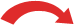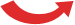# What is 2.325 as a fraction?

## What is 2.325 as a fraction?

2.325 is / as a fraction

#### How to convert decimals as fractions

 1.   What are fraction ? 2.   Steps to convert 2.325 to fraction ? 3.   How to read a fraction? 4.   Example of fractions in real life - 1 5.   Example of fractions in real life - 2

#### What are fraction ?

Word “fraction” is derived from the Latin word fractus. It simply means how many parts of a whole we have. A fraction consists of a numerator, a slash and denominator. For example in the fraction 3/4, 3 is the numerator and 4 is the denominator.

#### Steps to convert 2.325 to fraction ?

Steps:
• Step 1: Write down the decimal divided by 1, like this: decimal 1
• Step 2: Multiply both top and bottom by 10 for every number after the decimal point. (For example, if there are two numbers after the decimal point, then use 100, if there are three then use 1000, etc.)
• Step 3: Simplify (or reduce) the fraction
• ### Example: Convert 2.325 to a fraction

Step 1: Write down 2.325 divided by 1:

2.325 1

Step 2: Multiply both top and bottom by 1000 (because there are 3 digits after the decimal point so that is 1000:
 × 10002.325 1 = 1000× 1000

(Do you see how it turns the top number into a whole number?)

Step 3: Simplify the Fraction:
 × 10001000 =